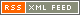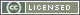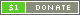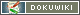# Logic Synthesis

I am interested in logic synthesis algorithms, and specifically in high performance algorithms for the solution of the P-equivalence problem for Boolean functions and related problems.

Two boolean functions are P-equivalent is there is a permutation of the input variables that leads from the first to the second. Finding the permutation, if it exists, is a time consuming step of logic synthesis algorithms, i.e. it is used in finding which combination of existing library logic cells can be used to map a given Boolean function.

We have designed a variant of the fastest known P-equivalence matching algorithm that runs in five times less than the baseline.

## People involved

• Prof. Donatella Sciuto
• Giovanni Agosta
• Francesco Bruschi
• Gerardo Pelosi